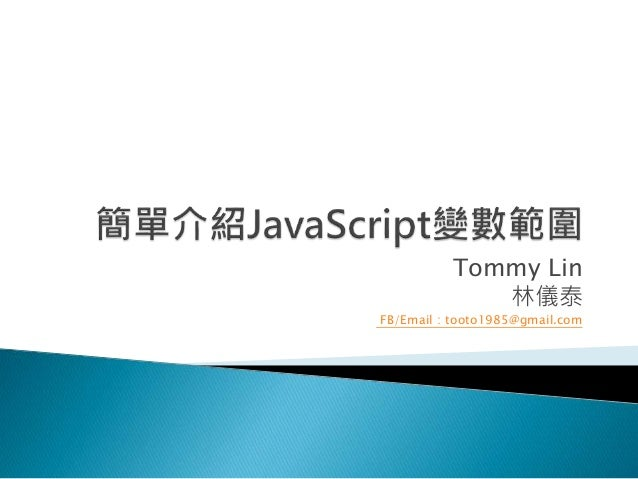Successfully reported this slideshow.
We use your LinkedIn profile and activity data to personalize ads and to show you more relevant ads. You can change your ad preferences anytime.Upcoming SlideShare
×

簡單介紹JavaScript變數範圍

959 views

Published on

• Full Name
Comment goes here.

Are you sure you want to Yes No
Your message goes here• Be the first to comment

簡單介紹JavaScript變數範圍

1. 1. Tommy Lin 林儀泰 FB/Email：tooto1985@gmail.com
2. 2.  限制被宣告的變數有效存取範圍 ◦ 函式內可以存取函式外的變數
3. 3. 存取函式外層變數 var a=100; function run() { var b=100; console.log(a); } run();
4. 4. 存取函式外層變數 var a=100; function run() { var b=100; console.log(a); //100 } run();
5. 5.  限制被宣告的變數有效存取範圍 ◦ 函式內可以存取函式外的變數 ◦ 函式外不可存取函式內的變數
6. 6. 存取函式內層變數 var a=100; function run() { var b=100; } run(); console.log(b);
7. 7. 存取函式內層變數 var a=100; function run() { var b=100; } run(); console.log(b); //undefined
8. 8.  限制被宣告的變數有效存取範圍 ◦ 函式內可以存取函式外的變數 ◦ 函式外不可存取函式內的變數  以函式來界定存取範圍(ES5)
9. 9. 函式界定範圍 var a=0; function run() { if(true) { var a=1; } console.log(a); } run(); console.log(a);
10. 10. 函式界定範圍 var a=0; function run() { if(true) { var a=1; } console.log(a); } run(); console.log(a); //0
11. 11. 函式界定範圍 var a=0; function run() { if(true) { var a=1; } console.log(a); //1 } run(); console.log(a); //0
12. 12.  限制被宣告的變數有效存取範圍 ◦ 函式內可以存取函式外的變數 ◦ 函式外不可存取函式內的變數  以函式來界定存取範圍(ES5) ◦ ES6支援以區塊界定範圍的宣告let
13. 13. 區塊界定範圍(ES6) function run() { var a = 0; if(true) { let a=1; console.log(a); } console.log(a); } run();
14. 14. 區塊界定範圍(ES6) function run() { var a = 0; if(true) { let a=1; console.log(a); //1 } console.log(a); } run();
15. 15. 區塊界定範圍(ES6) function run() { var a = 0; if(true) { let a=1; console.log(a); //1 } console.log(a); //0 } run();
16. 16.  限制被宣告的變數有效存取範圍 ◦ 函式內可以存取函式外的變數 ◦ 函式外不可存取函式內的變數  以函式來界定存取範圍(ES5) ◦ ES6支援以區塊界定範圍的宣告let  必須是套崁函式並非引用函式
17. 17. 套崁函式 var a = 100; function run() { var b = 200; function todo() { console.log(b); } todo(); console.log(a); } run();
18. 18. 套崁函式 var a = 100; function run() { var b = 200; function todo() { console.log(b); //200 } todo(); console.log(a); } run();
19. 19. 套崁函式 var a = 100; function run() { var b = 200; function todo() { console.log(b); //200 } todo(); console.log(a); //100 } run();
20. 20. 引用函式 var a = 100; function todo() { console.log(b); } function run() { var b = 200; todo(); console.log(a); } run();
21. 21. 引用函式 var a = 100; function todo() { console.log(b); } function run() { var b = 200; todo(); console.log(a); //100 } run();
22. 22. 引用函式 var a = 100; function todo() { console.log(b); //undefined } function run() { var b = 200; todo(); console.log(a); //100 } run();
23. 23.  內層可以存取外層  外層不可以存取內層  函式界定存取範圍  區塊界定存取範圍需使用let宣告(ES6)  必須是套崁函式才可存取
24. 24.  https://developer.mozilla.org/en- US/docs/Web/JavaScript/Reference/Statements/var  https://developer.mozilla.org/en- US/docs/Web/JavaScript/Reference/Statements/let